Prealgebra

# 4.1Visualize Fractions

Prealgebra4.1 Visualize Fractions

### Learning Objectives

By the end of this section, you will be able to:
• Understand the meaning of fractions
• Model improper fractions and mixed numbers
• Convert between improper fractions and mixed numbers
• Model equivalent fractions
• Find equivalent fractions
• Locate fractions and mixed numbers on the number line
• Order fractions and mixed numbers

### Be Prepared 4.1

Before you get started, take this readiness quiz.

1. Simplify: $5·2+1.5·2+1.$
If you missed this problem, review Example 2.8.
2. Fill in the blank with $<<$ or $>:−2__−5>:−2__−5$
If you missed this problem, review Example 3.2.

### Understand the Meaning of Fractions

Andy and Bobby love pizza. On Monday night, they share a pizza equally. How much of the pizza does each one get? Are you thinking that each boy gets half of the pizza? That’s right. There is one whole pizza, evenly divided into two parts, so each boy gets one of the two equal parts.

In math, we write $1212$ to mean one out of two parts.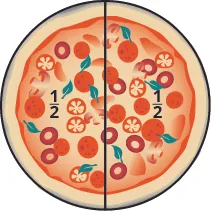On Tuesday, Andy and Bobby share a pizza with their parents, Fred and Christy, with each person getting an equal amount of the whole pizza. How much of the pizza does each person get? There is one whole pizza, divided evenly into four equal parts. Each person has one of the four equal parts, so each has $1414$ of the pizza.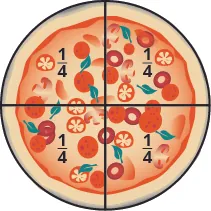On Wednesday, the family invites some friends over for a pizza dinner. There are a total of $1212$ people. If they share the pizza equally, each person would get $112112$ of the pizza.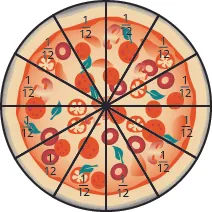### Fractions

A fraction is written $ab,ab,$ where $aa$ and $bb$ are integers and $b≠0.b≠0.$ In a fraction, $aa$ is called the numerator and $bb$ is called the denominator.

A fraction is a way to represent parts of a whole. The denominator $bb$ represents the number of equal parts the whole has been divided into, and the numerator $aa$ represents how many parts are included. The denominator, $b,b,$ cannot equal zero because division by zero is undefined.

In Figure 4.2, the circle has been divided into three parts of equal size. Each part represents $1313$ of the circle. This type of model is called a fraction circle. Other shapes, such as rectangles, can also be used to model fractions.

Figure 4.2

### Manipulative Mathematics

Doing the Manipulative Mathematics activity Model Fractions will help you develop a better understanding of fractions, their numerators and denominators.

What does the fraction $2323$ represent? The fraction $2323$ means two of three equal parts.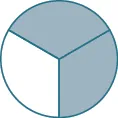### Example 4.1

Name the fraction of the shape that is shaded in each of the figures.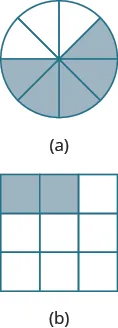### Try It 4.1

Name the fraction of the shape that is shaded in each figure: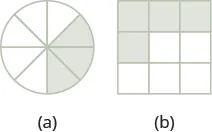### Try It 4.2

Name the fraction of the shape that is shaded in each figure: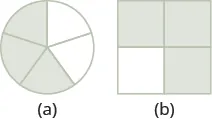### Example 4.2

Shade $3434$ of the circle.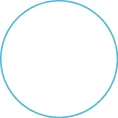### Try It 4.3

Shade $6868$ of the circle.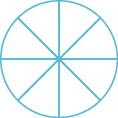### Try It 4.4

Shade $2525$ of the rectangle.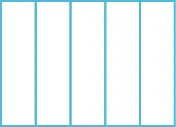In Example 4.1 and Example 4.2, we used circles and rectangles to model fractions. Fractions can also be modeled as manipulatives called fraction tiles, as shown in Figure 4.3. Here, the whole is modeled as one long, undivided rectangular tile. Beneath it are tiles of equal length divided into different numbers of equally sized parts.

Figure 4.3

We’ll be using fraction tiles to discover some basic facts about fractions. Refer to Figure 4.3 to answer the following questions:

 How many $1212$ tiles does it take to make one whole tile? It takes two halves to make a whole, so two out of two is $22=1.22=1.$ How many $1313$ tiles does it take to make one whole tile? It takes three thirds, so three out of three is $33=1.33=1.$ How many $1414$ tiles does it take to make one whole tile? It takes four fourths, so four out of four is $44=1.44=1.$ How many $1616$ tiles does it take to make one whole tile? It takes six sixths, so six out of six is $66=1.66=1.$ What if the whole were divided into $2424$ equal parts? (We have not shown fraction tiles to represent this, but try to visualize it in your mind.) How many $124124$ tiles does it take to make one whole tile? It takes $2424$ twenty-fourths, so $2424=1.2424=1.$

It takes $2424$ twenty-fourths, so $2424=1.2424=1.$

This leads us to the Property of One.

### Property of One

Any number, except zero, divided by itself is one.

$aa=1(a≠0)aa=1(a≠0)$

### Manipulative Mathematics

Doing the Manipulative Mathematics activity "Fractions Equivalent to One" will help you develop a better understanding of fractions that are equivalent to one

### Example 4.3

Use fraction circles to make wholes using the following pieces:

1. $44$ fourths
2. $55$ fifths
3. $66$ sixths

### Try It 4.5

Use fraction circles to make wholes with the following pieces: $33$ thirds.

### Try It 4.6

Use fraction circles to make wholes with the following pieces: $88$ eighths.

What if we have more fraction pieces than we need for $11$ whole? We’ll look at this in the next example.

### Example 4.4

Use fraction circles to make wholes using the following pieces:

1. $33$ halves
2. $88$ fifths
3. $77$ thirds

### Try It 4.7

Use fraction circles to make wholes with the following pieces: $55$ thirds.

### Try It 4.8

Use fraction circles to make wholes with the following pieces: $55$ halves.

### Model Improper Fractions and Mixed Numbers

In Example 4.4 (b), you had eight equal fifth pieces. You used five of them to make one whole, and you had three fifths left over. Let us use fraction notation to show what happened. You had eight pieces, each of them one fifth, $15,15,$ so altogether you had eight fifths, which we can write as $85.85.$ The fraction $8585$ is one whole, $1,1,$ plus three fifths, $35,35,$ or $135,135,$ which is read as one and three-fifths.

The number $135135$ is called a mixed number. A mixed number consists of a whole number and a fraction.

### Mixed Numbers

A mixed number consists of a whole number $aa$ and a fraction $bcbc$ where $c≠0.c≠0.$ It is written as follows.

$abcc≠0abcc≠0$

Fractions such as $54,32,55,54,32,55,$ and $7373$ are called improper fractions. In an improper fraction, the numerator is greater than or equal to the denominator, so its value is greater than or equal to one. When a fraction has a numerator that is smaller than the denominator, it is called a proper fraction, and its value is less than one. Fractions such as $12,37,12,37,$ and $11181118$ are proper fractions.

### Proper and Improper Fractions

The fraction $abab$ is a proper fraction if $a and an improper fraction if $a≥b.a≥b.$

### Manipulative Mathematics

Doing the Manipulative Mathematics activity "Model Improper Fractions" and "Mixed Numbers" will help you develop a better understanding of how to convert between improper fractions and mixed numbers.

### Example 4.5

Name the improper fraction modeled. Then write the improper fraction as a mixed number.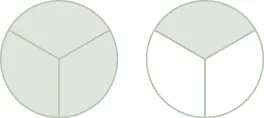### Try It 4.9

Name the improper fraction. Then write it as a mixed number.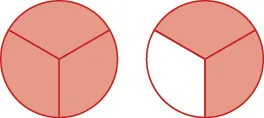### Try It 4.10

Name the improper fraction. Then write it as a mixed number.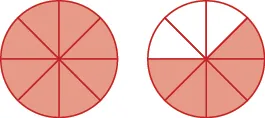### Example 4.6

Draw a figure to model $118.118.$

### Try It 4.11

Draw a figure to model $76.76.$

### Try It 4.12

Draw a figure to model $65.65.$

### Example 4.7

Use a model to rewrite the improper fraction $116116$ as a mixed number.

### Try It 4.13

Use a model to rewrite the improper fraction as a mixed number: $97.97.$

### Try It 4.14

Use a model to rewrite the improper fraction as a mixed number: $74.74.$

### Example 4.8

Use a model to rewrite the mixed number $145145$ as an improper fraction.

### Try It 4.15

Use a model to rewrite the mixed number as an improper fraction: $138.138.$

### Try It 4.16

Use a model to rewrite the mixed number as an improper fraction: $156.156.$

### Convert between Improper Fractions and Mixed Numbers

In Example 4.7, we converted the improper fraction $116116$ to the mixed number $156156$ using fraction circles. We did this by grouping six sixths together to make a whole; then we looked to see how many of the $1111$ pieces were left. We saw that $116116$ made one whole group of six sixths plus five more sixths, showing that $116=156.116=156.$

The division expression $116116$ (which can also be written as $611611$) tells us to find how many groups of $66$ are in $11.11.$ To convert an improper fraction to a mixed number without fraction circles, we divide.

### Example 4.9

Convert $116116$ to a mixed number.

### Try It 4.17

Convert the improper fraction to a mixed number: $137.137.$

### Try It 4.18

Convert the improper fraction to a mixed number: $149.149.$

### How To

#### Convert an improper fraction to a mixed number.

1. Step 1. Divide the denominator into the numerator.
2. Step 2. Identify the quotient, remainder, and divisor.
3. Step 3. Write the mixed number as quotient $remainderdivisorremainderdivisor$.

### Example 4.10

Convert the improper fraction $338338$ to a mixed number.

### Try It 4.19

Convert the improper fraction to a mixed number: $237.237.$

### Try It 4.20

Convert the improper fraction to a mixed number: $4811.4811.$

In Example 4.8, we changed $145145$ to an improper fraction by first seeing that the whole is a set of five fifths. So we had five fifths and four more fifths.

$55+45=9555+45=95$

Where did the nine come from? There are nine fifths—one whole (five fifths) plus four fifths. Let us use this idea to see how to convert a mixed number to an improper fraction.

### Example 4.11

Convert the mixed number $423423$ to an improper fraction.

### Try It 4.21

Convert the mixed number to an improper fraction: $357.357.$

### Try It 4.22

Convert the mixed number to an improper fraction: $278.278.$

### How To

#### Convert a mixed number to an improper fraction.

1. Step 1. Multiply the whole number by the denominator.
2. Step 2. Add the numerator to the product found in Step 1.
3. Step 3. Write the final sum over the original denominator.

### Example 4.12

Convert the mixed number $10271027$ to an improper fraction.

### Try It 4.23

Convert the mixed number to an improper fraction: $4611.4611.$

### Try It 4.24

Convert the mixed number to an improper fraction: $1113.1113.$

### Model Equivalent Fractions

Let’s think about Andy and Bobby and their favorite food again. If Andy eats $1212$ of a pizza and Bobby eats $2424$ of the pizza, have they eaten the same amount of pizza? In other words, does $12=24?12=24?$ We can use fraction tiles to find out whether Andy and Bobby have eaten equivalent parts of the pizza.

### Equivalent Fractions

Equivalent fractions are fractions that have the same value.

Fraction tiles serve as a useful model of equivalent fractions. You may want to use fraction tiles to do the following activity. Or you might make a copy of Figure 4.3 and extend it to include eighths, tenths, and twelfths.

Start with a $1212$ tile. How many fourths equal one-half? How many of the $1414$ tiles exactly cover the $1212$ tile?Since two $1414$ tiles cover the $1212$ tile, we see that $2424$ is the same as $12,12,$ or $24=12.24=12.$

How many of the $1616$ tiles cover the $1212$ tile?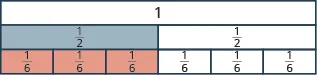Since three $1616$ tiles cover the $1212$ tile, we see that $3636$ is the same as $12.12.$

So, $36=12.36=12.$ The fractions are equivalent fractions.

### Manipulative Mathematics

Doing the activity "Equivalent Fractions" will help you develop a better understanding of what it means when two fractions are equivalent.

### Example 4.13

Use fraction tiles to find equivalent fractions. Show your result with a figure.

1. How many eighths equal one-half?
2. How many tenths equal one-half?
3. How many twelfths equal one-half?

### Try It 4.25

Use fraction tiles to find equivalent fractions: How many eighths equal one-fourth?

### Try It 4.26

Use fraction tiles to find equivalent fractions: How many twelfths equal one-fourth?

### Find Equivalent Fractions

We used fraction tiles to show that there are many fractions equivalent to $12.12.$ For example, $24,36,24,36,$ and $4848$ are all equivalent to $12.12.$ When we lined up the fraction tiles, it took four of the $1818$ tiles to make the same length as a $1212$ tile. This showed that $48=12.48=12.$ See Example 4.13.

We can show this with pizzas, too. Figure 4.4(a) shows a single pizza, cut into two equal pieces with $1212$ shaded. Figure 4.4(b) shows a second pizza of the same size, cut into eight pieces with $4848$ shaded.

Figure 4.4

This is another way to show that $1212$ is equivalent to $48.48.$

How can we use mathematics to change $1212$ into $48?48?$ How could you take a pizza that is cut into two pieces and cut it into eight pieces? You could cut each of the two larger pieces into four smaller pieces! The whole pizza would then be cut into eight pieces instead of just two. Mathematically, what we’ve described could be written as: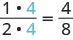These models lead to the Equivalent Fractions Property, which states that if we multiply the numerator and denominator of a fraction by the same number, the value of the fraction does not change.

### Equivalent Fractions Property

If $a,b,a,b,$ and $cc$ are numbers where $b≠0b≠0$ and $c≠0,c≠0,$ then

$ab=a·cb·cab=a·cb·c$

When working with fractions, it is often necessary to express the same fraction in different forms. To find equivalent forms of a fraction, we can use the Equivalent Fractions Property. For example, consider the fraction one-half.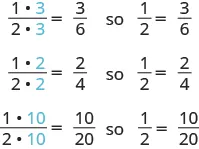So, we say that $12,24,36,12,24,36,$ and $10201020$ are equivalent fractions.

### Example 4.14

Find three fractions equivalent to $25.25.$

### Try It 4.27

Find three fractions equivalent to $35.35.$

### Try It 4.28

Find three fractions equivalent to $45.45.$

### Example 4.15

Find a fraction with a denominator of $2121$ that is equivalent to $27.27.$

### Try It 4.29

Find a fraction with a denominator of $2121$ that is equivalent to $67.67.$

### Try It 4.30

Find a fraction with a denominator of $100100$ that is equivalent to $310.310.$

### Locate Fractions and Mixed Numbers on the Number Line

Now we are ready to plot fractions on a number line. This will help us visualize fractions and understand their values.

### Manipulative Mathematics

Doing the Manipulative Mathematics activity "Number Line Part $33$" will help you develop a better understanding of the location of fractions on the number line.

Let us locate $15,45,3,313,74,92,5,15,45,3,313,74,92,5,$ and $8383$ on the number line.

We will start with the whole numbers $33$ and $55$ because they are the easiest to plot.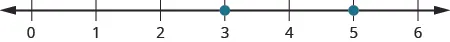The proper fractions listed are $1515$ and $45.45.$ We know proper fractions have values less than one, so $1515$ and $4545$ are located between the whole numbers $00$ and $1.1.$ The denominators are both $5,5,$ so we need to divide the segment of the number line between $00$ and $11$ into five equal parts. We can do this by drawing four equally spaced marks on the number line, which we can then label as $15,25,35,15,25,35,$ and $45.45.$

Now plot points at $1515$ and $45.45.$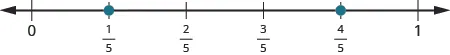The only mixed number to plot is $313.313.$ Between what two whole numbers is $313?313?$ Remember that a mixed number is a whole number plus a proper fraction, so $313>3.313>3.$ Since it is greater than $3,3,$ but not a whole unit greater, $313313$ is between $33$ and $4.4.$ We need to divide the portion of the number line between $33$ and $44$ into three equal pieces (thirds) and plot $313313$ at the first mark.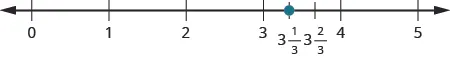Finally, look at the improper fractions $74,92,74,92,$ and $83.83.$ Locating these points will be easier if you change each of them to a mixed number.

$74=134,92=412,83=22374=134,92=412,83=223$

Here is the number line with all the points plotted.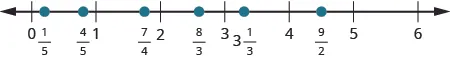### Example 4.16

Locate and label the following on a number line: $34,43,53,415,34,43,53,415,$ and $72.72.$

### Try It 4.31

Locate and label the following on a number line: $13,54,74,235,92.13,54,74,235,92.$

### Try It 4.32

Locate and label the following on a number line: $23,52,94,114,325.23,52,94,114,325.$

In Introduction to Integers, we defined the opposite of a number. It is the number that is the same distance from zero on the number line but on the opposite side of zero. We saw, for example, that the opposite of $77$ is $−7−7$ and the opposite of $−7−7$ is $7.7.$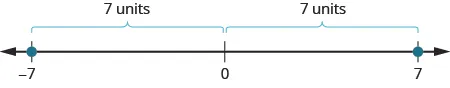Fractions have opposites, too. The opposite of $3434$ is $−34.−34.$ It is the same distance from $00$ on the number line, but on the opposite side of $0.0.$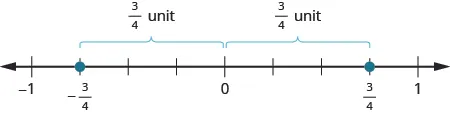Thinking of negative fractions as the opposite of positive fractions will help us locate them on the number line. To locate $−158−158$ on the number line, first think of where $158158$ is located. It is an improper fraction, so we first convert it to the mixed number $178178$ and see that it will be between $11$ and $22$ on the number line. So its opposite, $−158,−158,$ will be between $−1−1$ and $−2−2$ on the number line.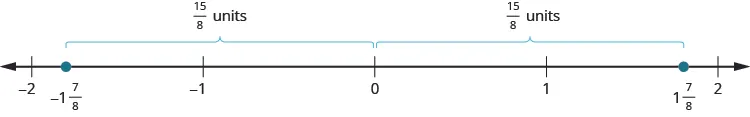### Example 4.17

Locate and label the following on the number line: $14,−14,113,−113,52,14,−14,113,−113,52,$ and $−52.−52.$

### Try It 4.33

Locate and label each of the given fractions on a number line:

$23,−23,214,−214,32,−3223,−23,214,−214,32,−32$

### Try It 4.34

Locate and label each of the given fractions on a number line:

$34,−34,112,−112,73,−7334,−34,112,−112,73,−73$

### Order Fractions and Mixed Numbers

We can use the inequality symbols to order fractions. Remember that $a>ba>b$ means that $aa$ is to the right of $bb$ on the number line. As we move from left to right on a number line, the values increase.

### Example 4.18

Order each of the following pairs of numbers, using $<<$ or $>:>:$

1. $−23____−1−23____−1$
2. $−312____−3−312____−3$
3. $−37____−38−37____−38$
4. $−2____−169−2____−169$

### Try It 4.35

Order each of the following pairs of numbers, using $<<$ or $>:>:$

1. $−13__−1−13__−1$
2. $−112__−2−112__−2$
3. $−23__−13−23__−13$
4. $−3__−73−3__−73$

### Try It 4.36

Order each of the following pairs of numbers, using $<<$ or $>:>:$

1. $−3__−175−3__−175$
2. $−214__−2−214__−2$
3. $−35__−45−35__−45$
4. $−4__−103−4__−103$

### Section 4.1 Exercises

#### Practice Makes Perfect

In the following exercises, name the fraction of each figure that is shaded.

1.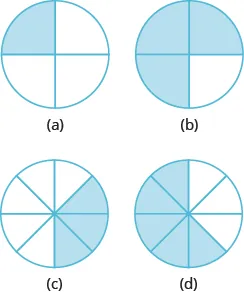2.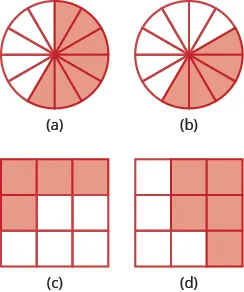In the following exercises, shade parts of circles or squares to model the following fractions.

3.

$1 2 1 2$

4.

$1 3 1 3$

5.

$3 4 3 4$

6.

$2 5 2 5$

7.

$5 6 5 6$

8.

$7 8 7 8$

9.

$5 8 5 8$

10.

$7 10 7 10$

In the following exercises, use fraction circles to make wholes, if possible, with the following pieces.

11.

$33$ thirds

12.

$88$ eighths

13.

$77$ sixths

14.

$44$ thirds

15.

$77$ fifths

16.

$77$ fourths

In the following exercises, name the improper fractions. Then write each improper fraction as a mixed number.

17.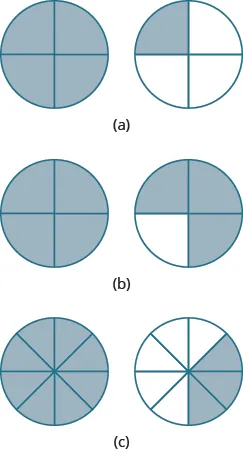18.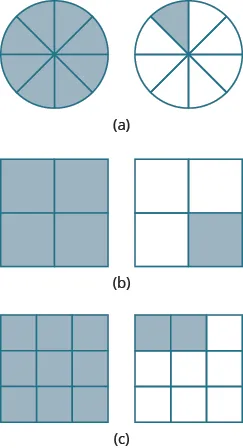19.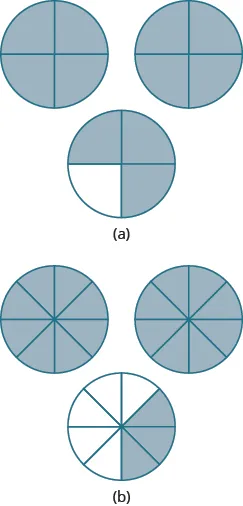In the following exercises, draw fraction circles to model the given fraction.

20.

$3 3 3 3$

21.

$4 4 4 4$

22.

$7 4 7 4$

23.

$5 3 5 3$

24.

$11 6 11 6$

25.

$13 8 13 8$

26.

$10 3 10 3$

27.

$9 4 9 4$

In the following exercises, rewrite the improper fraction as a mixed number.

28.

$3 2 3 2$

29.

$5 3 5 3$

30.

$11 4 11 4$

31.

$13 5 13 5$

32.

$25 6 25 6$

33.

$28 9 28 9$

34.

$42 13 42 13$

35.

$47 15 47 15$

In the following exercises, rewrite the mixed number as an improper fraction.

36.

$1 2 3 1 2 3$

37.

$1 2 5 1 2 5$

38.

$2 1 4 2 1 4$

39.

$2 5 6 2 5 6$

40.

$2 7 9 2 7 9$

41.

$2 5 7 2 5 7$

42.

$3 4 7 3 4 7$

43.

$3 5 9 3 5 9$

In the following exercises, use fraction tiles or draw a figure to find equivalent fractions.

44.

How many sixths equal one-third?

45.

How many twelfths equal one-third?

46.

How many eighths equal three-fourths?

47.

How many twelfths equal three-fourths?

48.

How many fourths equal three-halves?

49.

How many sixths equal three-halves?

In the following exercises, find three fractions equivalent to the given fraction. Show your work, using figures or algebra.

50.

$1 4 1 4$

51.

$1 3 1 3$

52.

$3 8 3 8$

53.

$5 6 5 6$

54.

$2 7 2 7$

55.

$5 9 5 9$

In the following exercises, plot the numbers on a number line.

56.

$2 3 , 5 4 , 12 5 2 3 , 5 4 , 12 5$

57.

$1 3 , 7 4 , 13 5 1 3 , 7 4 , 13 5$

58.

$1 4 , 9 5 , 11 3 1 4 , 9 5 , 11 3$

59.

$7 10 , 5 2 , 13 8 , 3 7 10 , 5 2 , 13 8 , 3$

60.

$2 1 3 , −2 1 3 2 1 3 , −2 1 3$

61.

$1 3 4 , −1 3 5 1 3 4 , −1 3 5$

62.

$3 4 , − 3 4 , 1 2 3 , −1 2 3 , 5 2 , − 5 2 3 4 , − 3 4 , 1 2 3 , −1 2 3 , 5 2 , − 5 2$

63.

$2 5 , − 2 5 , 1 3 4 , −1 3 4 , 8 3 , − 8 3 2 5 , − 2 5 , 1 3 4 , −1 3 4 , 8 3 , − 8 3$

In the following exercises, order each of the following pairs of numbers, using $<<$ or $>.>.$

64.

$−1 __ − 1 4 −1 __ − 1 4$

65.

$−1 __ − 1 3 −1 __ − 1 3$

66.

$−2 1 2 __ − 3 −2 1 2 __ − 3$

67.

$−1 3 4 __ − 2 −1 3 4 __ − 2$

68.

$− 5 12 __ − 7 12 − 5 12 __ − 7 12$

69.

$− 9 10 __ − 3 10 − 9 10 __ − 3 10$

70.

$−3 __ − 13 5 −3 __ − 13 5$

71.

$−4 __ − 23 6 −4 __ − 23 6$

#### Everyday Math

72.

Music Measures A choreographed dance is broken into counts. A $1111$ count has one step in a count, a $1212$ count has two steps in a count and a $1313$ count has three steps in a count. How many steps would be in a $1515$ count? What type of count has four steps in it?

73.

Music Measures Fractions are used often in music. In $4444$ time, there are four quarter notes in one measure.

1. How many measures would eight quarter notes make?
2. The song “Happy Birthday to You” has $2525$ quarter notes. How many measures are there in “Happy Birthday to You?”
74.

Baking Nina is making five pans of fudge to serve after a music recital. For each pan, she needs $1212$ cup of walnuts.

1. How many cups of walnuts does she need for five pans of fudge?
2. Do you think it is easier to measure this amount when you use an improper fraction or a mixed number? Why?

#### Writing Exercises

75.

Give an example from your life experience (outside of school) where it was important to understand fractions.

76.

Explain how you locate the improper fraction $214214$ on a number line on which only the whole numbers from $00$ through $1010$ are marked.

#### Self Check

After completing the exercises, use this checklist to evaluate your mastery of the objectives of this section.

If most of your checks were:

…confidently. Congratulations! You have achieved the objectives in this section. Reflect on the study skills you used so that you can continue to use them. What did you do to become confident of your ability to do these things? Be specific.

…with some help. This must be addressed quickly because topics you do not master become potholes in your road to success. In math, every topic builds upon previous work. It is important to make sure you have a strong foundation before you move on. Who can you ask for help? Your fellow classmates and instructor are good resources. Is there a place on campus where math tutors are available? Can your study skills be improved?

…no—I don’t get it! This is a warning sign and you must not ignore it. You should get help right away or you will quickly be overwhelmed. See your instructor as soon as you can to discuss your situation. Together you can come up with a plan to get you the help you need.

Order a print copy

As an Amazon Associate we earn from qualifying purchases.